# Ion

## Key Stage 4

### Meaning

An Ion is a particle that has a different number of protons to electrons.

 Atom Ion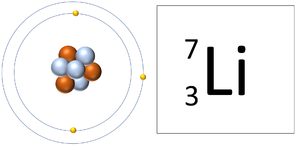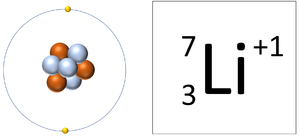A Lithium atom has 3 protons and 3 electrons so it is neutral. A Lithium ion has 3 protons and 2 electrons so it has a positive charge. It now has a full Outer Shell.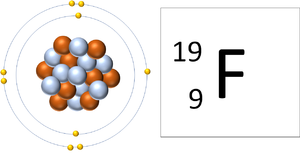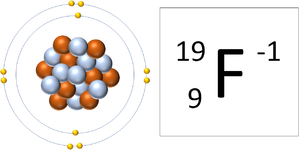A Fluorine atom has 9 protons and 9 electrons so it is neutral. A Fluorine ion has 9 protons and 10 electrons so it is negative charge. It now has a full Outer Shell.
 A Lithium Ion A Fluorine Ion A Boron Ion An Oxygen Ion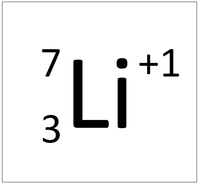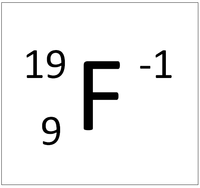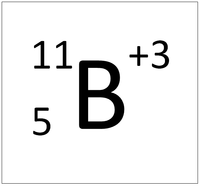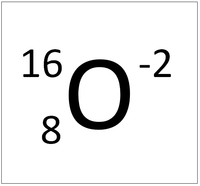This ion has an Atomic Number (Z) of 3 and a Relative Atomic Charge (Q) of +1. Number of electrons = A - Q Number of electrons = 3 - 1 Number of electrons = 2 This ion has an Atomic Number (Z) of 9 and a Relative Atomic Charge (Q) of -1. Number of electrons = A - Q Number of electrons = 9 - (-1) Number of electrons = 10 This ion has an Atomic Number (Z) of 5 and a Relative Atomic Charge (Q) of +3. Number of electrons = A - Q Number of electrons = 5 - 3 Number of electrons = 2 This ion has an Atomic Number (Z) of 8 and a Relative Atomic Charge (Q) of -2. Number of electrons = A - Q Number of electrons = 8 - (-2) Number of electrons = 10